# Greatest Integer and Fractional Part Functions

Go back to  'Functions'

The greatest integer less than or equal to a number x, or the floor of x, is represented as $$\left[ x \right]$$. For example:

$\begin{array}{l}\left[ {3.1} \right] = 3, &\left[ {2.999} \right] = 2\\\left[ { - 2.7} \right] = - 3, &\left[ { - \sqrt 2 } \right] = - 2\\\left[ 4 \right] = 4, & \left[ { - 7} \right] = - 7\end{array}$

Thus, if x is a number between successive integers n and $$n + 1$$,then $$\left[ x \right] = n$$. If is any integer, then $$\left[ x \right] = x$$.

Using this concept, we can define the greatest integer function, or the floor function, as follows:

$f\left( x \right) = \left[ x \right]$

Clearly, the input variable can take on any real value. However, the output will always be an integer. Also, all integers will occur in the output set. Thus, the domain of this function is  $$\mathbb{R}$$ , while its range will be  $$\mathbb{Z}$$.

Next, let us consider the fractional part of any number x. This is the difference between x and its greatest integer part, $$\left[ x \right]$$:

$\left\{ x \right\} = x - \left[ x \right]$

For example,

$\begin{array}{l}\left\{ {3.1} \right\} = 3.1 - \left[{3.1} \right] = 0.1\\\left\{ { - 2.7} \right\} = - 2.7 -\left[ { - 2.7} \right] = 0.3\end{array}$

Note that the fractional part will always be non-negative, as x will always be greater than (or equal to) $$\left[ x \right]$$. If x is an integer,then its fractional part will be 0.

Using the concept of the fractional part, we can define the fractional part function as follows:

$f\left( x \right) = \left\{ x \right\}$

For any real number x, its fractional part can be calculated. Thus, the domain of this function is $$\mathbb{R}$$. Now, let us determine its range. If x is an integer, then its fractional part will be 0. If x is a non-integer, let n and $$n + 1$$ be the integers between which x lies. We will have $$\left\{ x \right\} = x - n$$, which means that$$\left\{ x \right\}$$ must be greater than 0 but smaller than 1: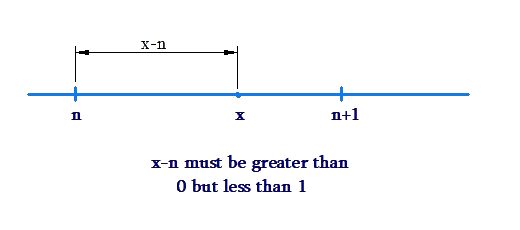We conclude that the range of the fractional part function is $$\left[ {0,1}\right)$$.

Now, we will plot the graphs of these two functions. First, consider $$f\left( x\right) = \left[ x \right]$$. If x is an integer, then the value of f will be x itself. If x is a non-integer, then the value of x will be the integer just before x.

For example, for all numbers lying in the interval $$\left[ {0,1} \right)$$, the value of f will be 0. For the entire interval $$\left[ {1,2} \right)$$, f will take the value 1. For the interval $$\left[ { - 1,0}\right)$$, f will take the value $$- 1$$. And so on. These observations lead us to the following graph: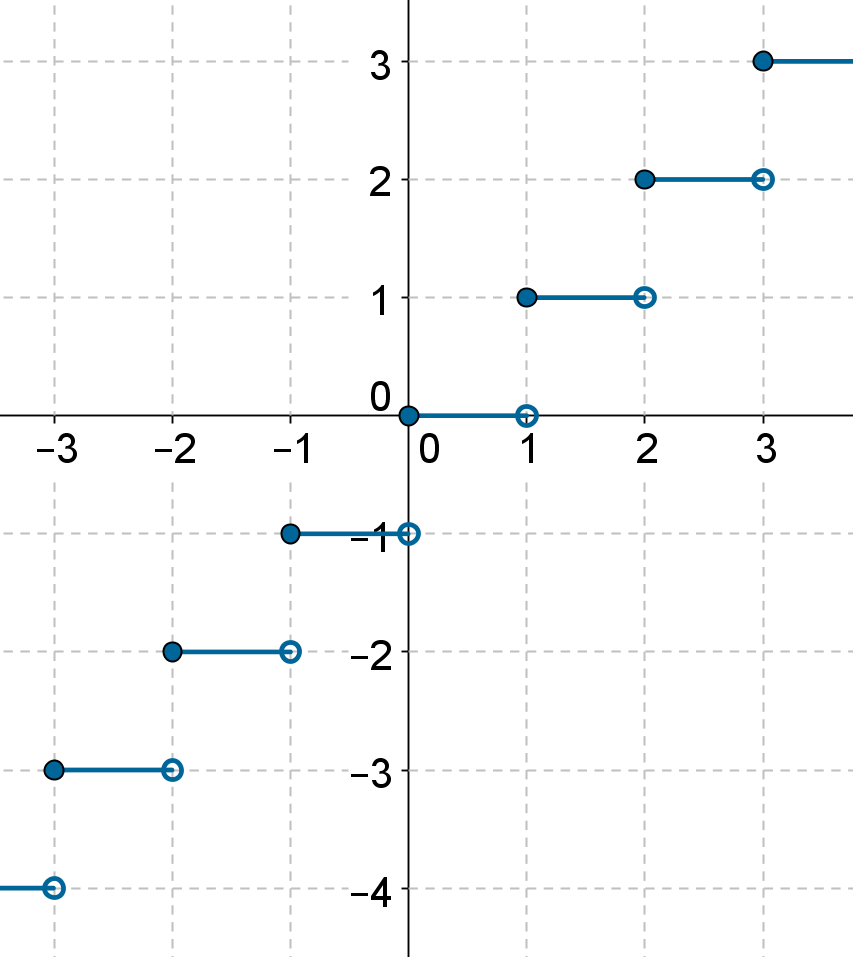The function has a constant value between any two integers. As soon as the next integer comes, the function value jumps by one unit. Carefully note the solid and hollow dots. For example, there is a hollow dot at $$\left( {1,0} \right)$$and a solid dot at $$\left( {1,1} \right)$$.This means that the value of f at $$x =1$$ is 1 (and not 0).

Now, we will graph the function $$g\left( x \right) = \left\{ x \right\}$$. Let us first start with $$x = 0$$. For this value of x, $$\left\{ x \right\} = 0$$.Now, as x is increased in the interval $$\left({0,1} \right)$$, the fractional part also increases, and has the same value as x. However, as soon as x takes the value 1, the fractional part goes back to 0. Let us plot the graph of g in only the interval $$\left[ {0,1} \right]$$: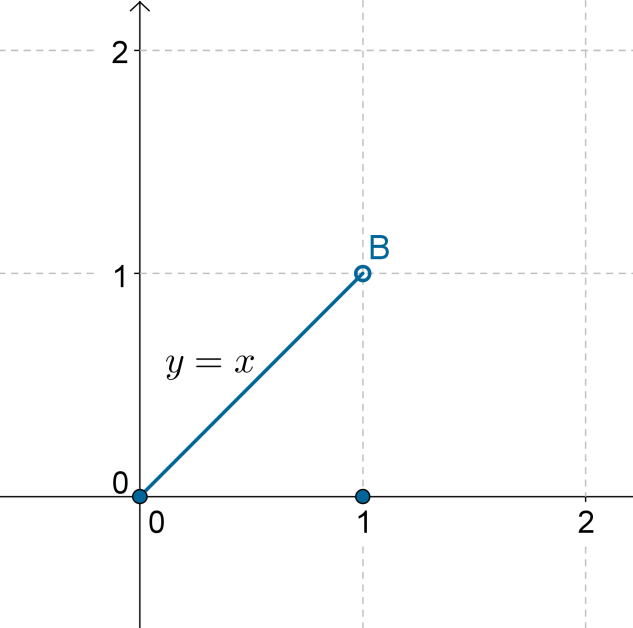Observe how the graph is the same as the line $$y = x$$ in the interval $$\left( {0,1} \right)$$, and then jumps back to 0 when $$x = 1$$.

Now, as x increases beyond 1,the fractional part will start to rise again (in the same manner as in the interval $$\left( {0,1} \right)$$), and will fall back to 0 when $$x = 2$$: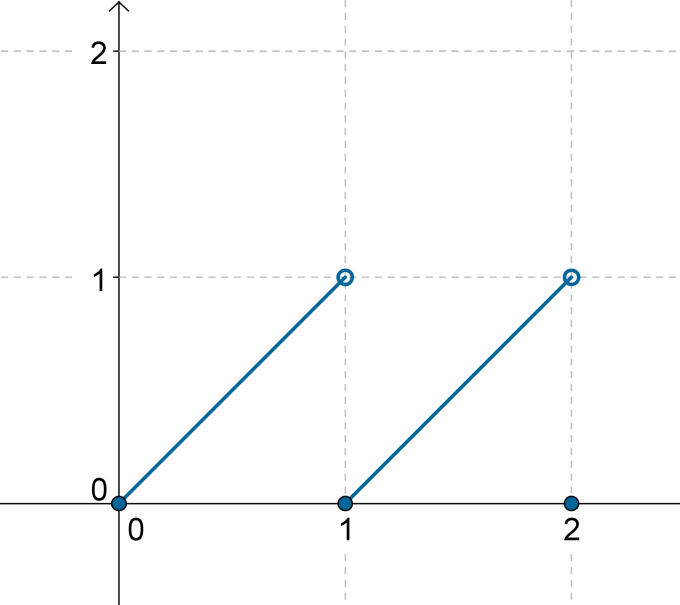Extending this, we obtain the graph of $$g\left( x \right) = \left\{ x \right\}$$over  $$\mathbb{R}$$ as follows: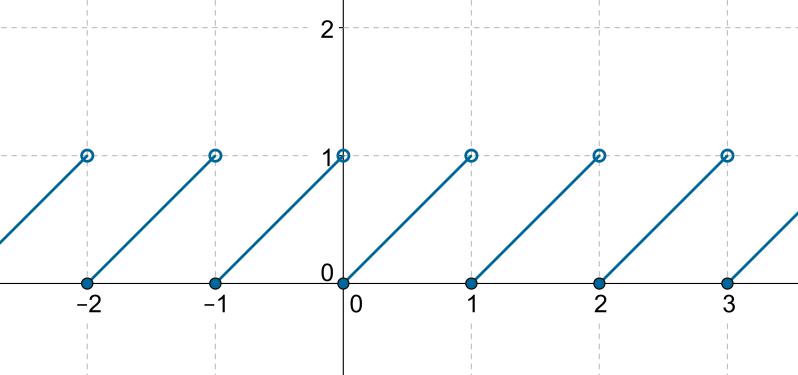From the graph, it is evident that the range of the fractional part function is $$\left[{0,1} \right)$$.

Example 1: What is the domain of (a) $$f\left( x \right) =\frac{1}{{\left[ x \right]}}$$ and   (b)    $$g\left(x \right) = \frac{1}{{\left\{ x \right\}}}$$?

Solution: (a) The denominator should not be 0, that is, $$\left[ x \right] \ne 0$$.The greatest integer part of a number is 0 if that number lies in the interval $$\left[ {0,1} \right)$$. Thus, to obtain our domain, this interval must be excluded from the set of real numbers. This means that the domain of f    is $$\mathbb{R}- \left[ {0,1} \right)$$.

(b) Once again, the denominator should not be 0, which means that $$\left\{ x\right\} \ne 0$$. When is the fractional part of a number equal to 0?Only when that number is an integer! Thus, the domain of g is  $$\mathbb{R}-\mathbb{Z}$$ , that is, all integers have been excluded from the set of real numbers.

Functions
Functions
grade 10 | Questions Set 2
Functions
Functions
grade 10 | Questions Set 1